# High School Physics : Understanding Normal Force

## Example Questions

### Example Question #1 : Understanding Normal Force

Which law proves that the normal force on an object will always be perpendicular to the surface it is on and that the formula will be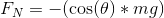?

None of these laws

Newton's first law

The second law of thermodynamics

Newton's second law

Newton's third law

Newton's third law

Explanation:

Newton's third law says that for every force, there is an equal and opposite force: for an object on a non-inclined plane, the force of gravity is straight down and the normal force is straight up.

(For non-inclined planes,still works, just use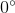for your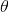as the cosine ofis.)

In non-mathematical terms, the normal force is what keeps us from sinking into the ground: we experience the force of gravity and yet we aren't sucked into the Earth's crust. Why? Because the object we stand upon provides an equal but opposite force on our feet.

This principle still applies to objects on an inclined plane. As the angle of the plane increases, the force of gravity is divided. Gravity causes the object to travel downward and horizontally along the plane's surface. The normal force must still counteract the downward portion of gravity, but will be less than the total gravitational force since the object is sliding horizontally. This requires us to use the cosine term to reduce the total normal force. Eventually, if the angle of incline increases enough, we would be taking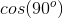, which is zero; if the object is in free fall (the plane is vertical), then there is no normal force.

### Example Question #2 : Understanding Normal Force

An object is lying at rest on a table. If the object has a mass of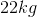, what is the normal force acting upon the object?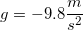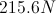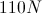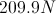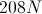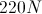Explanation:

The normal force on an object at rest on a flat surface is equal to the gravitational force on that object. In equation form, that means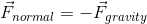.

Use Newton's second law (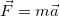) to solve for the gravitational force, using acceleration due to gravity as your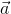.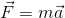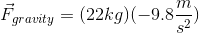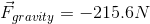As normal force is the opposite of the gravitational force, we can see that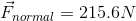.

### Example Question #3 : Understanding Normal Force

A man attempts to lift a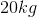box with a force of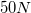. What is the normal force acting on the box while the man is lifting?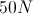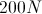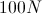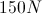Explanation:

Looking at our given values, we can see that each term only has one significant figure, since zeroes in front of the decimal are not significant. We can immediately determine that our answer will also have one significant figure however, to maintain accuracy, we will only round for precision after the final calculation.

The normal force acting on box is equal and opposite to the weight of the box. When the box is on a level surface, this term is equal to the force of gravity.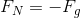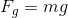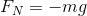Use our given mass and the acceleration due to gravity to solve for the normal force of the box.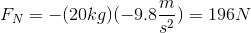This value is equal to the total upward force on the box to counter its weight. When the man is lifting the box, he accommodates part of this upward force, lessening the normal force.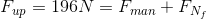Find the final normal force by subtracting the force from the man from the total upward force.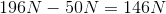Our given numbers only allow for one significant figure, so we must round down.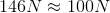### Example Question #4 : Understanding Normal Force

If an object at rest on a table weighs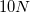, what force does the table exert on the object?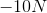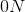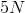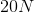Explanation:

Normal force is defined as the force that a surface exerts on an object. If the object is at rest, net force on the object is equal to zero; therefore, the downward force (weight) must be equal to the upward force (normal force).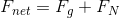Since weight acts in the downward direction it will be negative. The total sum of the forces must be zero, in order for the object to be at rest.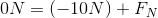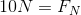This is also in accordance with Newton's third law, which suggests that the normal force will be equal and opposite the force of weight.

### Example Question #5 : Understanding Normal Force

A wooden cube is resting on a table. The cube has a mass of 100kg. What is the magnitude and direction of the normal force?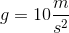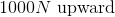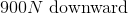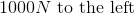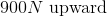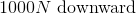Explanation:

Normal force is the force that the table pushes onto the block. If the force on the block from gravity is pushing the block downward, then the normal force has to push straight upward to keep the block at rest. Since the block is not moving, these two forces must be equal and opposite to produce a net force of zero.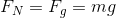Since normal force is pushing upward, it will be positive. (The force of gravity would be negative).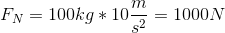### Example Question #6 : Understanding Normal Force

A box sits on a ramp at an incline of 30 degrees. If the box has a weight of 50N, what is the normal force experienced by the box?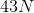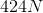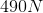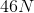Explanation:

The correct answer is 43N. Since the box is on an incline, normal force balances with the component of gravity that is perpendicular to the surface of the incline.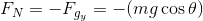Note that the normal force is in the upward (positive) direction, while gravitational acceleration and the force of gravity are in the downward (negative) direction.

We are given the weight of the box (note that it will be downward and, therefore, negative):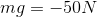Use this to solve for the normal force.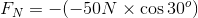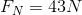### Example Question #7 : Understanding Normal Force

Normal force always acts __________.

opposite of gravity

along the vertical axis

perpendicular to the contact surface

directly upwards